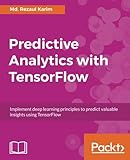# Predictive Analytics with TensorFlowPredictive Analytics with TensorFlow:Implement deep learning principles to predict valuable insights using TensorFlow

by:Md. Rezaul Karim
ISBN-10 书号：1788398920
ISBN-13 书号：9781788398923
Release Finelybook 出版日期：2017-11-02
pages 页数：522

Book Description
Predictive analytics discovers hidden patterns from structured and unstructured data for automated decision-making in business intelligence.
This book will help you build, tune, and deploy predictive models with TensorFlow in three main sections. The first section covers linear algebra, statistics, and probability theory for predictive modeling.
The second section covers developing predictive models via supervised (classification and regression) and unsupervised (clustering) algorithms. It then explains how to develop predictive models for NLP and covers reinforcement learning algorithms. Lastly, this section covers developing a factorization machines-based recommendation system.
The third section covers deep learning architectures for advanced predictive analytics, including deep neural networks and recurrent neural networks for high-dimensional and sequence data. Finally, convolutional neural networks are used for predictive modeling for emotion recognition, image classification, and sentiment analysis.
Contents
1:BASIC PYTHON AND LINEAR ALGEBRA FOR PREDICTIVE ANALYTICS
2:STATISTICS, PROBABILITY, AND INFORMATION THEORY FOR PREDICTIVE MODELING
3:FROM DATA TO DECISIONS – GETTING STARTED WITH TENSORFLOW
4:PUTTING DATA IN PLACE – SUPERVISED LEARNING FOR PREDICTIVE ANALYTICS
5:CLUSTERING YOUR DATA – UNSUPERVISED LEARNING FOR PREDICTIVE ANALYTICS
6:PREDICTIVE ANALYTICS PIPELINES FOR NLP
7:USING DEEP NEURAL NETWORKS FOR PREDICTIVE ANALYTICS
8:USING CONVOLUTIONAL NEURAL NETWORKS FOR PREDICTIVE ANALYTICS
9:USING RECURRENT NEURAL NETWORKS FOR PREDICTIVE ANALYTICS
10:RECOMMENDATION SYSTEMS FOR PREDICTIVE ANALYTICS
11:USING REINFORCEMENT LEARNING FOR PREDICTIVE ANALYTICS
What You Will Learn
Get a solid and theoretical understanding of linear algebra, statistics, and probability for predictive modeling
Develop predictive models using classification, regression, and clustering algorithms
Develop predictive models for NLP
Learn how to use reinforcement learning for predictive analytics
Factorization Machines for advanced recommendation systems
Get a hands-on understanding of deep learning architectures for advanced predictive analytics
Learn how to use deep Neural Networks for predictive analytics
See how to use recurrent Neural Networks for predictive analytics
Convolutional Neural Networks for emotion recognition, image classification, and sentiment analysis
Authors
Md. Rezaul Karim
Md. Rezaul Karim is a research scientist at Fraunhofer FIT, Germany. He is also a PhD candidate at RWTH Aachen University, Germany. Before joining FIT, he worked as a researcher at the Insight Center for Data Analytics, Ireland. He was a lead engineer at Samsung Electronics, Korea. He has 9 years’ R&D experience with C++, Java, R, Scala, and Python. He has published research papers on bioinformatics, big data, and deep learning. He has practical working experience with Spark, Zeppelin, Hadoop, Keras, Scikit-Learn, TensorFlow, Deeplearning4j, MXNet, and H2O.

### 觉得文章有用就打赏一下

#### 支付宝扫一扫打赏#### 微信扫一扫打赏